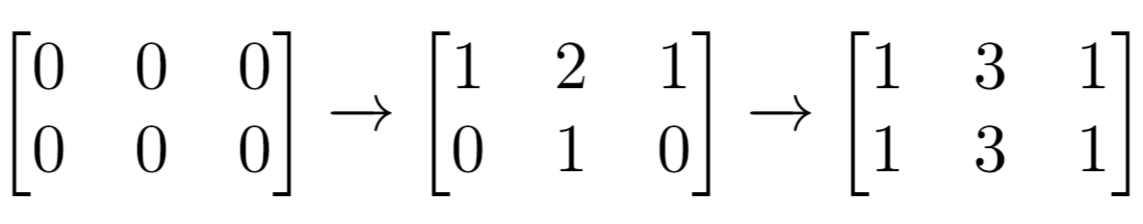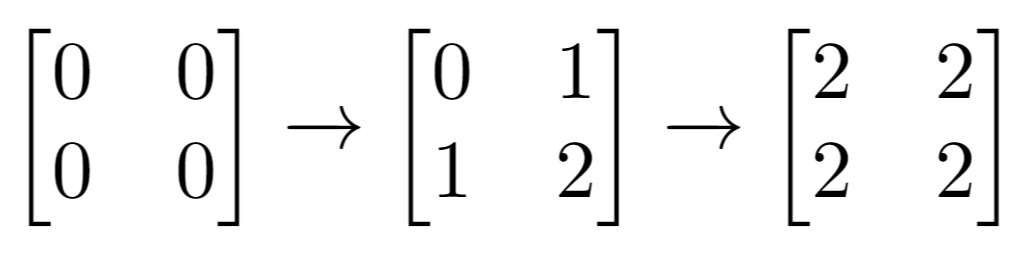##### Welcome to Subscribe On Youtube

Formatted question description: https://leetcode.ca/all/1252.html

# 1252. Cells with Odd Values in a Matrix (Easy)

Given n and m which are the dimensions of a matrix initialized by zeros and given an array indices where indices[i] = [ri, ci]. For each pair of [ri, ci] you have to increment all cells in row ri and column ci by 1.

Return the number of cells with odd values in the matrix after applying the increment to all indices.

Example 1:Input: n = 2, m = 3, indices = [[0,1],[1,1]]
Output: 6
Explanation: Initial matrix = [[0,0,0],[0,0,0]].
After applying first increment it becomes [[1,2,1],[0,1,0]].
The final matrix will be [[1,3,1],[1,3,1]] which contains 6 odd numbers.


Example 2:Input: n = 2, m = 2, indices = [[1,1],[0,0]]
Output: 0
Explanation: Final matrix = [[2,2],[2,2]]. There is no odd number in the final matrix.


Constraints:

• 1 <= n <= 50
• 1 <= m <= 50
• 1 <= indices.length <= 100
• 0 <= indices[i] < n
• 0 <= indices[i] < m

Related Topics:
Array

## Solution 1.

// OJ: https://leetcode.com/problems/cells-with-odd-values-in-a-matrix/
// Time: O(MN)
// Space: O(M+N)
class Solution {
public:
int oddCells(int n, int m, vector<vector<int>>& indices) {
vector<int> row(n, 0), col(m, 0);
for (auto &v : indices) {
row[v]++;
col[v]++;
}
int ans = 0;
for (int i = 0; i < n; ++i) {
for (int j = 0; j < m; ++j) {
if ((row[i] + col[j]) % 2) ans++;
}
}
return ans;
}
};

• class Solution {
public int oddCells(int n, int m, int[][] indices) {
int[] rowsCount = new int[n];
int[] columnsCount = new int[m];
for (int[] index : indices) {
int row = index, column = index;
rowsCount[row]++;
columnsCount[column]++;
}
int oddRows = 0, oddColumns = 0;
for (int rowCount : rowsCount) {
if (rowCount % 2 != 0)
oddRows++;
}
for (int columnCount : columnsCount) {
if (columnCount % 2 != 0)
oddColumns++;
}
return oddRows * (m - oddColumns) + oddColumns * (n - oddRows);
}
}

############

class Solution {
public int oddCells(int m, int n, int[][] indices) {
int[] row = new int[m];
int[] col = new int[n];
for (int[] e : indices) {
int r = e, c = e;
row[r]++;
col[c]++;
}
int ans = 0;
for (int i : row) {
for (int j : col) {
ans += (i + j) % 2;
}
}
return ans;
}
}

• // OJ: https://leetcode.com/problems/cells-with-odd-values-in-a-matrix/
// Time: O(MN)
// Space: O(M+N)
class Solution {
public:
int oddCells(int n, int m, vector<vector<int>>& indices) {
vector<int> row(n, 0), col(m, 0);
for (auto &v : indices) {
row[v]++;
col[v]++;
}
int ans = 0;
for (int i = 0; i < n; ++i) {
for (int j = 0; j < m; ++j) {
if ((row[i] + col[j]) % 2) ans++;
}
}
return ans;
}
};

• class Solution:
def oddCells(self, m: int, n: int, indices: List[List[int]]) -> int:
row =  * m
col =  * n
for r, c in indices:
row[r] += 1
col[c] += 1
return sum((i + j) % 2 for i in row for j in col)

############

# 1252. Cells with Odd Values in a Matrix
# https://leetcode.com/problems/cells-with-odd-values-in-a-matrix/

class Solution:
def oddCells(self, n: int, m: int, indices: List[List[int]]) -> int:
grid = [*m for _ in range(n)]

for r,c in indices:
for i in range(n):
for j in range(m):
if j == c:
grid[i][j] += 1
if i == r:
grid[i][j] += 1

return sum(1 for num in sum(grid,[]) if num & 1)


• func oddCells(m int, n int, indices [][]int) int {
row := make([]int, m)
col := make([]int, n)
for _, e := range indices {
r, c := e, e
row[r]++
col[c]++
}
ans := 0
for _, i := range row {
for _, j := range col {
ans += (i + j) % 2
}
}
return ans
}